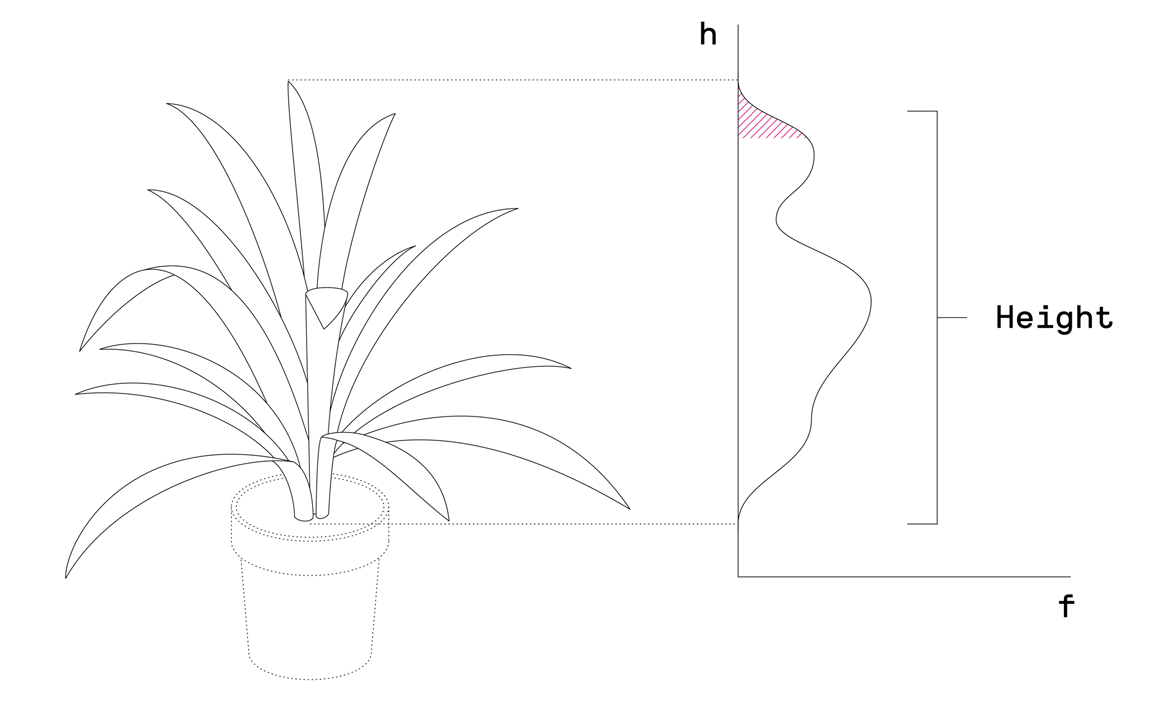Value Range: 0 –  ∞
Unit: mm

To calculate plant height PlantEye uses the distribution of elementary triangles  along the z-axis.  To do that first, the histogram along the z-axis is calculated. This histogram expresses how many elementary triangles are present in what height above ground.

After that, the histogram is cropped at both ends which means that a certain safety margin on the upper and lower end of the plants is removed to make the calculation more robust against single outliers. To finally calculate height, the top 10% of the plant are averaged and the height is calculated as the range from pot height up to this averaged value.